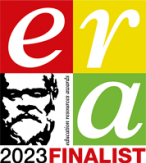# AQA GCSE (8525) SLR 11 – Boolean logic

This download provides you with a zip file which contains all the resources you will need to deliver this topic.

It includes:

• Student Learning Record Workbook (for use by students)
• Student Learning Record Answers (for use by teachers)
• End of Topic test and answers
• Lesson PowerPoint’s for every lesson
• Any associated answers or references sheets for class activities

This topic covers:

3.4.2 Boolean logic

• Construct truth tables for the following logic gates:
• NOT
• AND
• OR
• XOR
• Construct truth tables for simple logic circuits using combinations of NOT, AND, OR and XOR gates.
• Interpret the results of simple truth tables.
• Create, modify and interpret simple logic circuit diagrams.
• Students will only need to use NOT, AND, OR and XOR gates within logic circuits.
• Students will be expected to understand and use logic circuit symbols.
• Create and interpret simple Bodean expressions made up of NOT, AND, OR and XOR operations.
• Create the Bodean expression for a simple logic circuit.
• Create a logic circuit from a simple Bodean expression.Registered in England and Wales: 10442992

VAT Number: 290 9845 58

Telephone: 020 4519 3010